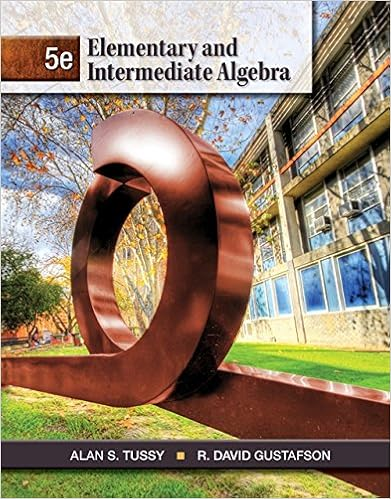# Day38class2 - Day 38/6.3 Math 1431 If you email me please...

• Notes
• 19

This preview shows page 1 - 6 out of 19 pages.

##### We have textbook solutions for you!
The document you are viewing contains questions related to this textbook.The document you are viewing contains questions related to this textbook.
Chapter 4 / Exercise 9
Elementary and Intermediate Algebra
Tussy/GustafsonExpert Verified
Day 38/6.3 Math 1431 If you email me, please put your class in the subject line. Have you registered for Test 4? Don’t forget the online quizzes. THINK:The following number has a rather special characteristic. What makes it unique? 8,549,176,320
##### We have textbook solutions for you!
The document you are viewing contains questions related to this textbook.The document you are viewing contains questions related to this textbook.
Chapter 4 / Exercise 9
Elementary and Intermediate Algebra
Tussy/GustafsonExpert Verified
Section 6.3 Volume by Shell Method
Popper 1. The region Rin the first quadrant is enclosed by the lines x = 0 and y = 5 and the curve y = x2+ 1. The volume of the solid generated when Ris revolved about the y-axis is
π54415
2. Let Rbe the region in the first quadrant bounded by the x-axis and the curve y = 2x – x2. The volume produced when Rrevolved about the x-axis is is
E) π8
3. Given the region in the first quadrant bounded by the function y = 4 – x2, set up the integral equation that finds the volume of the region when rotated about y = 0, using the disc/washer method. a. (29=-220V4xdxb. (29= π-220V4xdxc. (29= π--220V4xdxd. (29= π-20V4xdx2222
•••